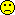## Mathematical wizard [Bioanalytics]

Hi Martin,

I really loved one of the examples from your last reference:
  STDEV(1,2,3) = 1   STDEV(90000001,90000002,90000003) = 0
which looks even more funny if you run this set (in M$-Excel 5.0 and 2000 – the only versions I have handy):  STDEV(0.90000001,0.90000002,0.90000003) = 0 STDEV(0.9000001,0.9000002,0.9000003) = 0.0000001 STDEV(0.900001,0.900002,0.900003) = 0.000001 STDEV(0.90001,0.90002,0.90003) = 0.00001 STDEV(0.9001,0.9002,0.9003) = 0.0001 STDEV(0.901,0.902,0.903) = 0.001 STDEV(0.91,0.92,0.93) = 0.01 STDEV(9.1,9.2,9.3) = 0.1 STDEV(91,92,93) = 1 STDEV(901,902,903) = 1 STDEV(9001,9002,9003) = 1 STDEV(90001,90002,90003) = 1 STDEV(900001,900002,900003) = 1 STDEV(9000001,9000002,9000003) = 1 STDEV(90000001,90000002,90000003) = 0 STDEV(9000000001,9000000002,9000000003) = 0 STDEV(90000000001,90000000002,90000000003) = 0 STDEV(900000000001,900000000002,900000000003) = 0 STDEV(9000000000001,9000000000002,9000000000003) = 131072 STDEV(90000000000001,90000000000002,90000000000003) = 0 STDEV(900000000000001,900000000000002,900000000000003) = 0R 2.8.1  sd(c(90000001,90000002,90000003)) = 1 STATISTICA 5.1H  St.Dev.(90000001,90000002,90000003) = 1 NCSS 2001  Standard Deviation (90000001,90000002,90000003) = 1 Maxima 5.15.0*  std1([90000001,90000002,90000003]); = 1 GNU Octave 3.0.3  std([90000001,90000002,90000003]) = 1 WinNonlin 5.2.1  STDEV(90000001;90000002;90000003) = 0 As you said, it’s advisable to use statistical software for statistical calculations (surprisingly PK software failed this simple test as well). Since this thread is in the Bioanalytics category: as far as I know Thermo’s Xcalibur software has only the options to store data in its own proprietary format or to export xls-files.But help is on the way. All (?) statistical software are able to import xls-files. • When it comes to numerical precision, it’s nice to have a clever algorithm. Maxima gives a standard deviation of 1 even for a set of {9·101,000+1, 9·101,000+2, 9·101,000+3}…If you don't believe me, run this code in Maxima: load(descriptive)$ std1(([9,9,9])*10^1000+([1,2,3]));

Dif-tor heh smusma 🖖
Helmut SchützThe quality of responses received is directly proportional to the quality of the question asked. 🚮
Science QuotesIng. Helmut Schütz